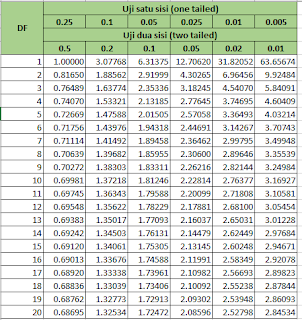-->

# Creating a T-Table Using Excel

Usually, when comparing t-test values ​​with t-counts, we always see the attachment of the t-test table in the Reference Book. but actually, we can make it on Microsoft Excel by using the Excel Formula "= TINV (Significance Value; Degree of Freedom)" or "= TINV (Significance Value, Degree of Freedom)" depending on the Excel settings used.

## Creating a T-Table Using Excel

The procedure for making this Statistical T Test Table is
First, prepare a table layout like the following,Second, Enter the formula in cell B5 = ---> = TINV (B \$ 4; \$ A5)
\$ captions are used to mark numbers or fixed letters and cannot be changed if
copy the formula by sliding it.
Third, copy the formula in cell B5 to all cells that you want to get the value of t table
Fourth, the table that will be obtained as below,Good luck